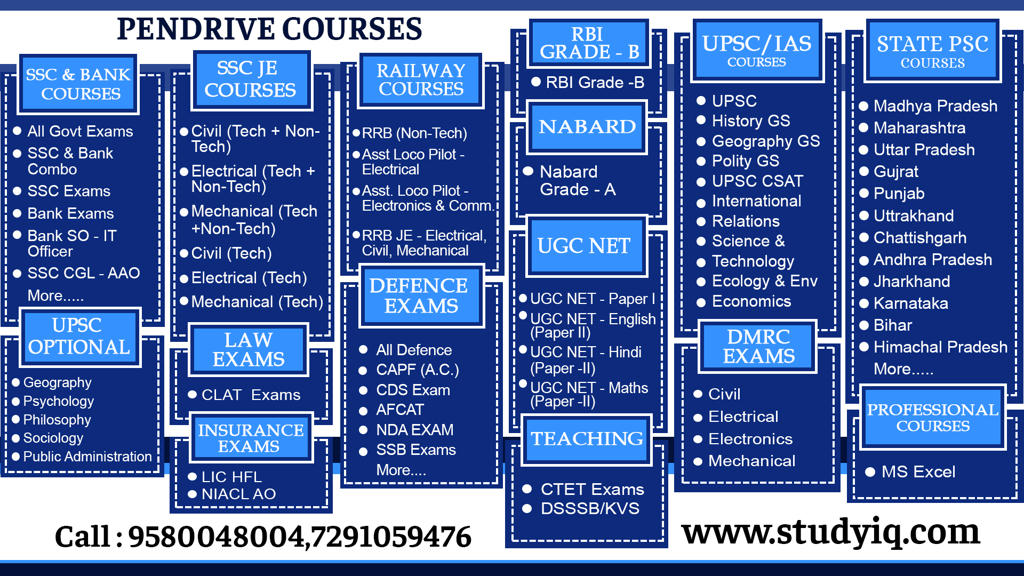# Profit and Loss Questions – Part 3 – Maths & Reasoning | PDF DownloadQ1. A man sells two articles for Rs 1710 he earns 10% loss on the first article and 25% profit on the second article if the CP of first article is equal to selling of II article find profit or loss

Q2.  A man purchased two articles for Rs 7500 shares at 20% profit and II at 50% less then find the amount of profit or loss if CP of first article is equal to selling price of second article

Q3. A person sold an article at rupees 144 in such a way that the profit percent and the CP are numerically the same find cost price of article

1) 80

2) 90

3) 100

4) 110

Q4. A person sold an article at rupees 96 in such a way that the profit percent and the CP and are numerically the same find cost price of article

1) 60

2) 70

3) 80

4) 90

Q5. A Sells a good to B at a profit of 16  2/3 % and B sell it to C at a profit of 9  1/11 % if she pays rupees to 5600 for it what was the cost price for

Q6. A sold an article to B at the profit of 33 1/3 % B sold it to C at a loss of 10% and C sold it to D at the profit of 16 2 /3 % if D paid rupees 4200 for it how much A paid to buy this article

Q7. A man sells two chair 120 rupees each, at one he get 25% profit and in another he get 25% loss so find his loss in rupees

Q8. A man sells two bull 8400 rupees each . In the end he get no profit or no loss. If he get 20% profit on on first then how much loss percent he get on second

Q9. After giving 20 percent discount a man still gains 10% profit find ratio of cost price and market

Q10. After giving 20 percent discount a man still gains 10% profit find ratio of cost price and market price PROFIT AND LOSS PART-3 RAHUL SIR

Q11.  price of a radio is 430 rupees the shopkeeper allows a discount of 10% and still gains 8% if no discount is allowed find his gain

Q12. A shopkeeper sells a TV set on discount of 20% of printed price and gains 10% , marked price was rupees 1100 then what was the cost price

Q13. A shopkeeper giving 10 percent discount still gains 20% profit find marked price whose cost price is Rs. 450

Q14. Tradesman marks his goods at such a price that after allowing a discount of 12 1/2 % for cash payment he makes a profit of 20% what is the Marked price of the article which cost him rupees 210

Q15. A dealer price of their goods is 25% more than purchase price and gives 10% discount for cash payment what will it profit

Q16. A dealer price of their goods is 40% more than purchase price and gives 20% discount for cash payment what will it profit or loss percent

Q17. Successively 14 2/7 % 11 1/9 % and 12 1/2 % of discount is equal to a single percentage discount

Q18. Successively 33 1/3 % and 12 1/2 % of discount is equal to a single percentage discount

Q19. The list price of the book ₹ 320 one retailer pays 244.80 after two successively discount of 10% and x% accordingly this value of x is PROFIT AND LOSS PART-3 RAHUL SIR

Q20.  The list price of the mixy ₹ 1600 one retailer pays 1224 after two successively discount of 10% and x% accordingly this value of x

Q21. The list price of a mobile phone is Rs. 1500 and a discount of 10% is proposed, then how many discount sheets should be given to a customer so that its actual value is Rs. 1242